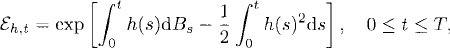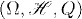# CHANGE OF MEASURE

The notion of change of measure has important applications in mathematical finance. In this chapter, we present some relevant concepts and theorems such as the Girsanov theorems.

# 37.1 Basic Concepts and Facts

Definition 37.1 (Exponential Process). Let. The exponential process given by h is defined to bewhere the Bt is a standard Brownian motion.

Theorem 37.1 (Novikov’s Theorem). Let {Xt : 0 ≤ tT} be a martingale, where T ≤ ∞. Let {Mt : 0 ≤ tT}be defined aswhereXt is the compensator of X2t (see Theorem 34.2). Suppose thatThen {Mt : 0 ≤ tT} is a continuous martingale.

Theorem 37.2 (Lévy Characterization of Brownian Motion). Let X = {Xt = (Xt(1),…, X(d)t) : t ≥ 0} be a continuous stochastic process on the probability spacewith the state space (R

Get Measure, Probability, and Mathematical Finance: A Problem-Oriented Approach now with the O’Reilly learning platform.

O’Reilly members experience live online training, plus books, videos, and digital content from nearly 200 publishers.